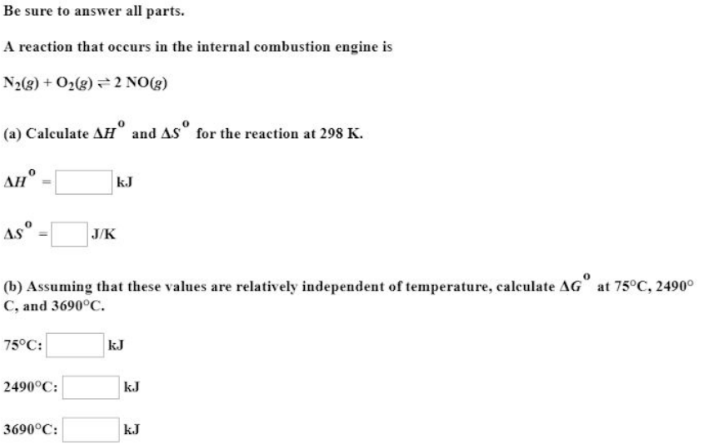# A reaction that occurs in the internal combustion engine is N2(g) + O2(g) <-> 2NO(g) (a) Calculate delta H^0 and delta S^0 for the reaction at 298 K. (b) Assuming that these values area relatively independent of temperature, calculate delta G^0 at 75 degrees Celsius, 2490 degrees Celsius, and 2690 degrees Celsius.